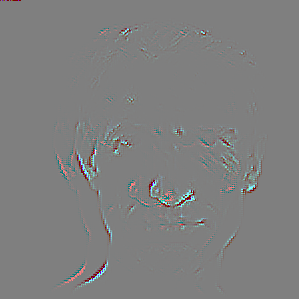# CPU and CUDA tensor to OpenCV Mat differ

When I run the model on cuda and on cpu in Libtorch I get different images while the tensor is almost the same (some numerical error?). To see whether the tensor are the same I subtract and then sum them which results in a small deviation of around 10e-5.

The resulting images, however, differ a lot. When I construct the image as follows:

``````    img = img.to(torch::kUInt8);
Mat imout(512,512,CV_8UC3);

memcpy((void*)imout.data, img.data_ptr(), sizeof(torch::kUInt8)*img.numel());
``````

The image is exactly the same as in Python. The cuda image, however, has 9 mini images as such:After some research I figured that the order of channels in memory may be different. I tried to construct my own OpenCV Mat by iterating over each element in order.

``````   Mat imout(512,512,CV_8UC3);
for(int i = 0; i<512; i++){
for(int j = 0; j<512; j++){
for(int k = 0; k<3; k++){
imout.at<uint8_t>(i,j,k) = img[i][j][k].item<uint8_t>();
}
}
}
``````

This results in a colour channel shifted, 3d glasses like, image when I run the model on cuda.How can I correctly turn a CUDA tensor to a Mat